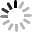## What exactly is dynamic range?

November 11, 2021

What exactly is dynamic range?

The concept of dynamic range often appears in engineering discussions. But what exactly does this term mean? How is it applied to electronic circuits and systems?

In the engineering field, we put more emphasis on accuracy and clarity. It is not easy to discuss technical details, mathematical relationships, and performance specifications. We can make tasks easier to manage and produce through careful use and thorough understanding of related terms.

A term often encountered by electrical engineers-"dynamic range". Before you continue reading, spend a minute trying to give a precise and comprehensive definition. It may be difficult, I hope the following article can help you understand this definition.

Static and dynamic range

As far as I know, the term "static scope" does not exist. But we can use it to clarify the nature of dynamic range.

"Range" refers to the area of change between two limits."Static" comes from a Greek verb related to standing or standing still. Therefore, the static range refers to an area of possible change in which the restriction is not affected by the need for active and unpredictable movement of input or output phenomena between the upper and lower limits.

"Dynamic" is essentially the opposite of "static"-describing things that are constantly moving and changing.For dynamic range, we still have upper and lower limits, but these upper limits apply to systems subject to continuous changes in input or output phenomena, and they capture the system's ability relative to operating conditions that reflect the dynamic nature of the relevant signal.

Considering the above information, in the field of electrical engineering, dynamic range specifies the range of possible or acceptable values that a dynamic signal can assume when it is delivered to or generated by a given system.Typical example-human vision

The human visual system has a remarkable ability to change the amount of input.We can see the ripples on the pond in the moonlight, and the white sails gleaming in the dazzling Mediterranean sun.The brightness range that the human eye is sensitive to is divided into 14 orders of magnitude: the lower limit is about 10-6 candelas per square meter (cd/m2), and the upper limit is about 108 cd/m2.

Does this mean that the dynamic range of the human visual system is 1014? No, because the brightness range mentioned above includes the sensitivity of cone cells and rod cells, and these two biological photoreceptors cannot work at the same time (the system actually needs It takes a long time to transition from high-brightness mode to low-brightness mode).Therefore, this range is not suitable for input signals that dynamically change between the upper and lower limits, thereby limiting the system to a specific set of operating conditions.

In order to determine the dynamic range of human light detection, we need to evaluate our ability to perceive the range of brightness when looking at a scene.If we adopt a stricter interpretation of the dynamic range, we are not allowed to change the pupil size, because this is equivalent to changing the upper or lower limit by increasing or decreasing the gain.

Calculation and expression of dynamic range

The dynamic range is actually a ratio: maximum signal level/minimum signal level.

Electrical engineers tend to use decibels to express large ratios (such as the gain of an op amp), and dynamic range is no exception.For example, if the dynamic range of a voltage monitoring system is 80db, the maximum detectable input level is 10,000 times larger than the minimum detectable input level.

In an optical system, the dynamic range is usually expressed as a stop.It refers to the increase or decrease of two factors. If the dynamic range of a digital camera is 8 lenses, then when the brightness of the brightest part divided by the brightness of the darkest part is less than or equal to 256 (=28), the scene can be reproduced well.The dB value is rounded to one decimal place, and the stop value is rounded to the nearest third stop point.

Dynamic range in electronic systems

The following provides examples of dynamic range in different types of circuits.

Digital-to-analog converter

If we consider the output, the dynamic range of the ADC is determined by the resolution.The smallest non-zero output value is 1, and the largest output value is (2N-1), where N is the number of bits of the generated digital word.Therefore, the dynamic output range of a 14-bit ADC is (214-1)=16383. This can also be expressed as 84.3 dB or 14 stops.

The dynamic range of the input signal is not so straightforward, because the lower limit is determined by the amount of noise in the analog waveform, and the noise is affected by environmental conditions or the gain setting of the variable gain amplifier before the ADC.When the upper limit of the reference voltage is 2.5 V and the noise floor is 1 mV, the dynamic range is 2.5/1×10-3≈68 dB.A special type of dynamic range called spurious-free dynamic range (SFDR) can be used to quantify the linearity of a circuit.

Image Sensor

In a CCD sensor, the upper limit of optical detection is the number of electrons that can be stored in a pixel, and the lower limit is the number of electrons related to dark noise and read noise.Assume that a pixel can hold 40,000 electrons. If a dark noise pixel generates 5 electrons at a given exposure time and receives an additional 10 electrons in the readout, the dynamic range is 40000 / (5 + 10) = 68.5 dB or about 11 stops.

RF Mixer

The mixer can perform frequency conversion, so it plays an important role in the design of the radio frequency system.When the mixer begins to exhibit unacceptable nonlinearity, the input power level is called the 1db compression point. This corresponds to the upper limit used to calculate the dynamic range, while the lower limit is the noise figure of the mixer.